Courses

# Test: Rotational Motion (Competition Level 2) - 1

## 25 Questions MCQ Test Physics Class 11 | Test: Rotational Motion (Competition Level 2) - 1

Description
This mock test of Test: Rotational Motion (Competition Level 2) - 1 for Class 11 helps you for every Class 11 entrance exam. This contains 25 Multiple Choice Questions for Class 11 Test: Rotational Motion (Competition Level 2) - 1 (mcq) to study with solutions a complete question bank. The solved questions answers in this Test: Rotational Motion (Competition Level 2) - 1 quiz give you a good mix of easy questions and tough questions. Class 11 students definitely take this Test: Rotational Motion (Competition Level 2) - 1 exercise for a better result in the exam. You can find other Test: Rotational Motion (Competition Level 2) - 1 extra questions, long questions & short questions for Class 11 on EduRev as well by searching above.
*Multiple options can be correct
QUESTION: 1

### ABCD is a square plate with centre O. The moments of inertia of the plate about the perpendicular axis through O is I and about the axes 1, 2, 3 & 4 are I1, I2, I3 & I4 respectively. It follows that :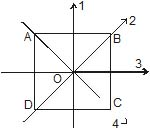Solution:

The perpendicular axis theorem states that the moment of inertia of a planar lamina about an axis perpendicular to the plane of the lamina is equal to the sum of the moments of inertia of the lamina about the two axes at right angles to each other, in its own plane intersecting each other at the point where the perpendicular axis passes through it.
Also as the given lamina is a square the axises through all the faces and all the diagonals are equal among themselves.

*Multiple options can be correct
QUESTION: 2

### A rod of weight w is supported by two parallel knife edges A and B and is in equilibrium in a horizontal position. The knives are at a distance d from each other. The centre of mass of the rod is at a distance x from A.

Solution:
*Multiple options can be correct
QUESTION: 3

### A block with a square base measuring axa and height h, is placed on an inclined plane. The coefficient of friction is m. The angle of inclination (q) of the plane is gradually increased. The block will

Solution:
*Multiple options can be correct
QUESTION: 4

A body is in equilibrium under the influence of a number of forces. Each force has a different line of action. The minimum number of forces required is

Solution:

If they are not parallel then we can assume a case where they are inclined at 120o to each other and that will cover all scenarios
If they are parallel then assume two forces acting upwards F1 and F2 that will balance the net torque and F3 acting downwards to balance out the net force
For the final case of 4 forces it will be a special case of the previous scenario. Assume F1 and F2 acting downwards and F3 and F4 acting upwards.

*Multiple options can be correct
QUESTION: 5

A block of mass m moves on a horizontal rough surface with initial velocity v. The height of the centre of mass of the block is h from the surface. Consider a point A on the surface

Solution:

The line of motion of the block passes through the center of mass and indicates the motion of center of mass. Hence, angular momentum about A is mvh initially. Since, the block is moving on a horizontal rough surface, due to  the frictional force the block will stop moving after some time. Hence, the velocity of the block decreases as time passes and due to this angular momentum is not conserved about A

*Multiple options can be correct
QUESTION: 6

Four point masses are fastened to the corners of a frame of negligible mass lying in the xy plane. Let w be the angular speed of rotation. Then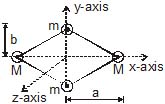Solution: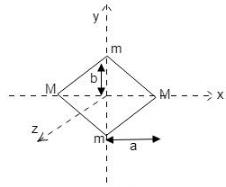Ix=2M(0)+2m(b2)=2mb2
KEx=(1/2)Ix ω2=mb2ω2
Iy=2M(a2)+2m(0)=2Ma2
KEy=(1/2)Iyω2=Ma2ω2
Iz=2M(a2)+2m(b2)=2(Ma2+mb2)
KEz=(1/2)Izω2=(Ma2+mb22

*Multiple options can be correct
QUESTION: 7

A particle falls freely near the surface of the earth. Consider a fixed point O (not vertically below the particle) on the ground.

Solution:
*Multiple options can be correct
QUESTION: 8

A man spinning in free space changes the shape of his body, eg. by spreading his arms or curling up. By doing this, he can change his

Solution:
1. If size changes then obviously MOI changes
2. By conservation of angular momentum it stays the same
3. L = Iw, since L is constant then if I changes w has to change
4. KE = L2/2I, to KE will also change
*Multiple options can be correct
QUESTION: 9

A ring rolls without slipping on the ground. Its centre C moves with a constant speed u. P is any point on the ring. The speed of P with respect to the ground is v.

Solution:
*Multiple options can be correct
QUESTION: 10

A disc of circumference s is at rest at a point A on a horizontal surface when a constant horizontal force begins to act on its centre. Between A and B there is sufficient friction to prevent slipping, and the surface is smooth to the right of B. AB = s. The disc moves from A to B in time T. To the right of B,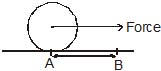Solution:
1. This is wrong because the linear acceleration will increase
2. By the previous argument this is correct
3. No, it will complete one rotation in time T
4. Since the acceleration will be greater the distance covered in the same time T will be greater than s
*Multiple options can be correct
QUESTION: 11

In the figure shown, the plank is being pulled to the right with a constant speed v. If the cylinder does not slip then :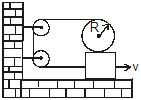Solution: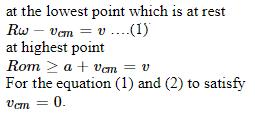So, Rw = v ⇒ w = v/R

*Multiple options can be correct
QUESTION: 12

If a cylinder is rolling down the incline with sliding

Solution:

For sliding Vcm > Rw
If the rate of change of Vcm is greater than rate of change of angular velocity i.e. dVcm/dt > dw/dt then pure rolling will not happen
But if dVcm/dt < dw/dt then pure rolling may start

*Multiple options can be correct
QUESTION: 13

Which of the following statements are correct

Solution:
*Multiple options can be correct
QUESTION: 14

Consider a sphere of mass `m' radius `R' doing pure rolling motion on a rough surface having velocity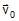as shown in the Figure. It makes an elastic impact with the smooth wall and moves back and starts pure rolling after some time again.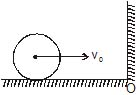Solution:

Taking angular momentum about the point PP
0−mV0R=Iω + mVR
⇒ (2/5)mR2×V0/R−mV0R=2/5mR2×V/R+mVR
⇒V=−3V0/7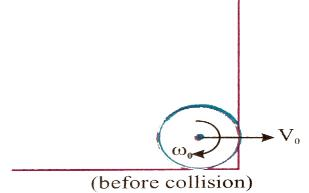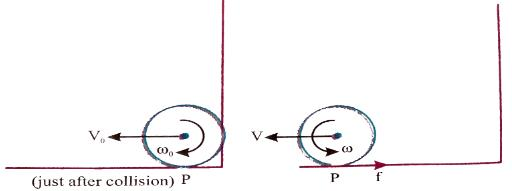*Multiple options can be correct
QUESTION: 15

A solid sphere, a hollow sphere and a disc, all having same mass and radius, are placed at the top of an incline and released. The friction coefficients between the objects and the incline are same and not sufficient to allow pure rolling. The smallest kinetic energy at the bottom of the incline will be achieved by

Solution:

Using Newton’s second law of motion, we get
Mgsinθ – μMgcosθ = Ma
So, a = gsinθ – μgcosθ which means that acceleration is independent of mass and since s = ½ at2 so t is same.

*Multiple options can be correct
QUESTION: 16

Fig. shows a smooth inclined plane fixed in a car accelerating on a horizontal road. The angle of incline q is related to the acceleration a of the car as a = g tan q. If the sphere is set in pure rotation on the incline.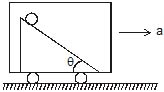Solution: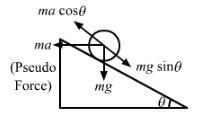From the free body diagram of sphere, we have:
Net force on the sphere along the incline,
Fnet = mgsinθ − macosθ    …(i)
On putting a = gtanθ in equation (i), we get:
Fnet = 0
Therefore, if the sphere is set in pure rolling on the incline, it will continue pure rolling.

QUESTION: 17

The figure shows an isosceles triangular plate of mass M and base L. The angle at the apex is 90°. The apex lies at the origin and the base is parallel to X - axis.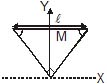The moment of inertia of the plate about the z-axis is

Solution:

By the perpendicular axis theorem, moment of inertia of z axis = moment of inertia of y axis + x axis
ml2/12 + ml2/12
ml2/6

QUESTION: 18

The moment of inertia of the plate about the x-axis is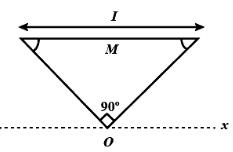Solution: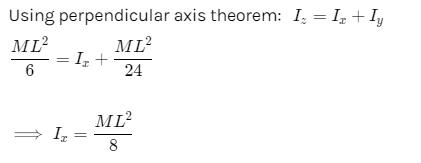QUESTION: 19

The moment of inertia of the plate about its base parallel to the x-axis isSolution:
QUESTION: 20

The moment of inertia of the plate about the y-axis isSolution:
*Multiple options can be correct
QUESTION: 21

A uniform rod is fixed to a rotating turntable so that its lower end is on the axis of the turntable and it makes an angle of 20° to the vertical. (The rod is thus rotating with uniform angular velocity about a vertical axis passing through one end.) If the turntable is rotating clockwise as seen from above.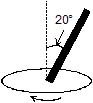What is the direction of the rod's angular momentum vector (calculated about its lower end)

Solution:
*Multiple options can be correct
QUESTION: 22

Is there a torque acting on it, and if so in what direction?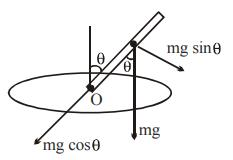Solution: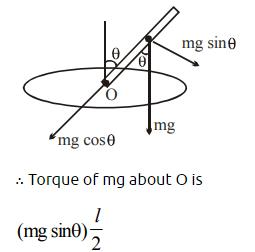*Multiple options can be correct
QUESTION: 23

A cylinder and a ring of same mass M and radius R are placed on the top of a rough inclined plane of inclination q. Both are released simultaneously from the same height h.

Choose the correct statement(s) related to the motion of each body

Solution:
*Multiple options can be correct
QUESTION: 24

Identify the correct statement(s)

Solution:
QUESTION: 25

When these bodies roll down to the foot of the inclined plane, then

Solution: